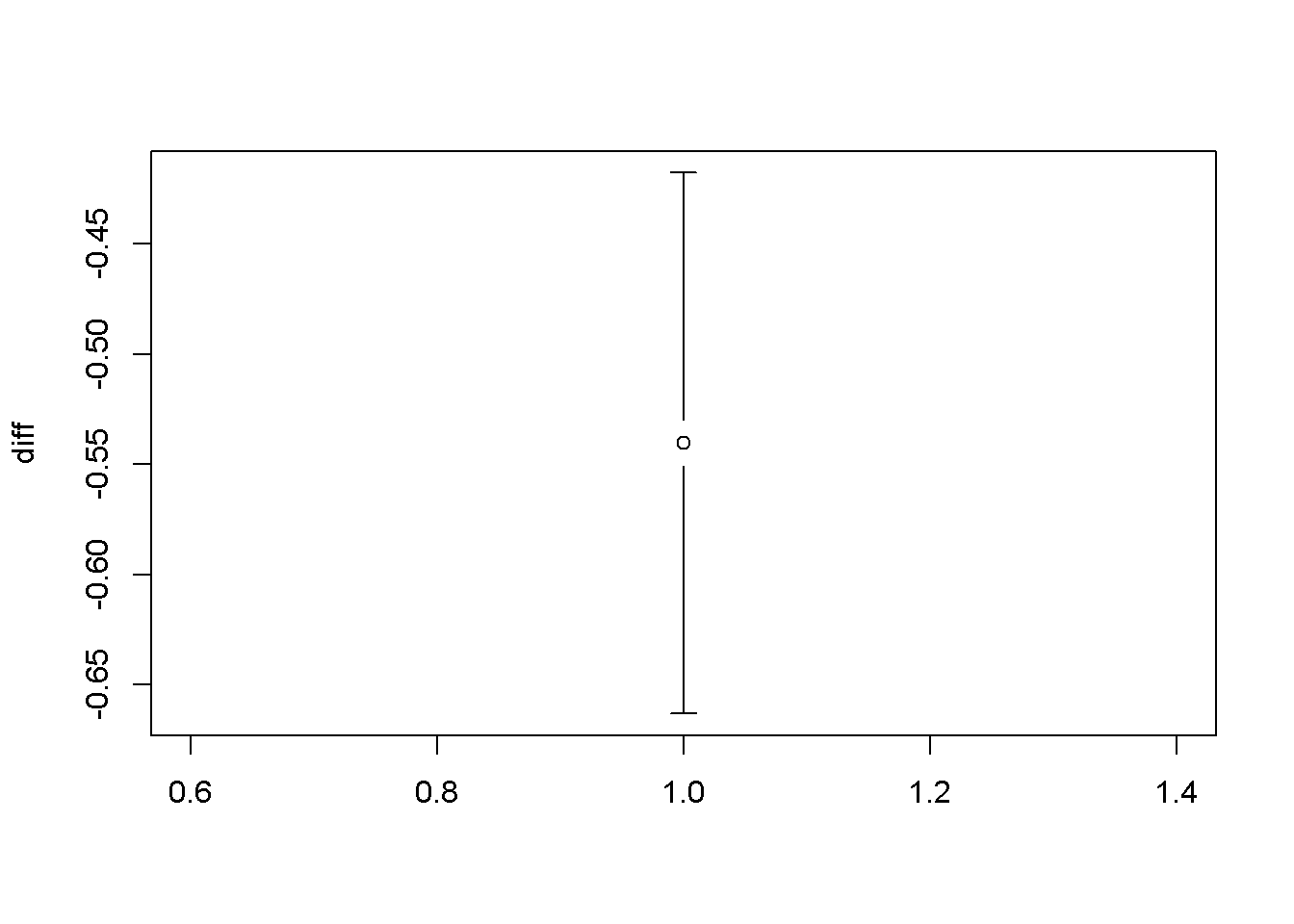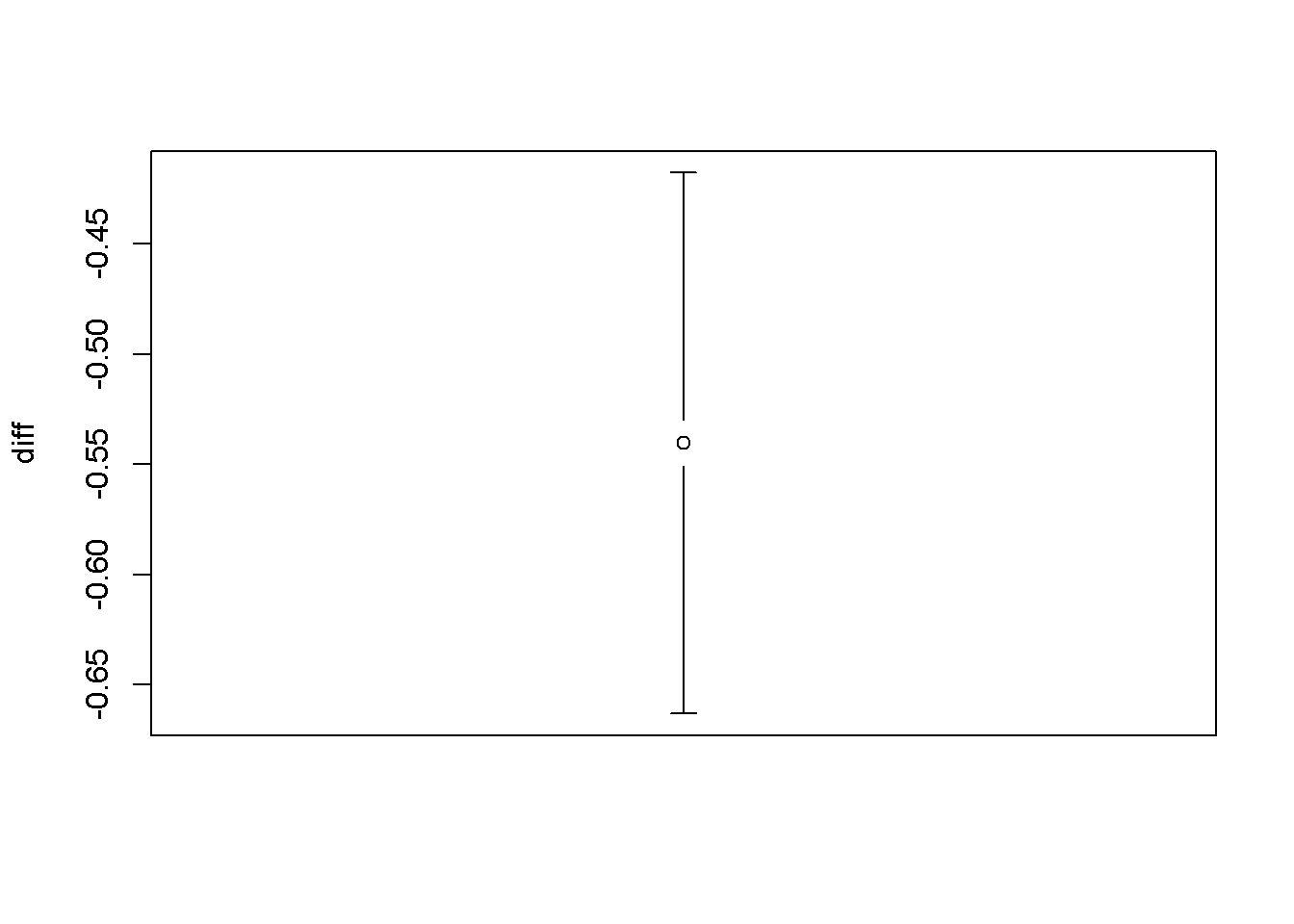Making effect size plots for t-test results

• I always try to emphasize making effect size plots.
• In a publication I would probably not devout an entire plot just to the effect size of a t-test, but I think making a simple graph like this is good practice.
• Here I go through the steps I would do to make a polished plot

Functions and arguements used

• t.test
• gplots::plotCI()
• par(xaxt=‘n’): removes axis ticks and numbers’
• par(mar = c(x,y,z,t)): change size of margins (bottom, left, top, right)
• mtext: adds texts to margins; can be adjusted to show up anywhere
• abline
• col: change color of a line
• lty: change line type

Data: Fisher’s cat data

library(MASS)
data(cats)
summary(cats)
##  Sex         Bwt             Hwt
##  F:47   Min.   :2.000   Min.   : 6.30
##  M:97   1st Qu.:2.300   1st Qu.: 8.95
##         Median :2.700   Median :10.10
##         Mean   :2.724   Mean   :10.63
##         3rd Qu.:3.025   3rd Qu.:12.12
##         Max.   :3.900   Max.   :20.50

t-test: are male cats bigger

t.test(Bwt ~ Sex, data = cats)
##
##  Welch Two Sample t-test
##
## data:  Bwt by Sex
## t = -8.7095, df = 136.84, p-value = 8.831e-15
## alternative hypothesis: true difference in means is not equal to 0
## 95 percent confidence interval:
##  -0.6631268 -0.4177242
## sample estimates:
## mean in group F mean in group M
##        2.359574        2.900000
• Yup

Make and effect size plot of this t-test

Save t-test output

t.test.out <- t.test(Bwt ~ Sex, data = cats)

Calculate difference of means

• This is our effect size
means <- t.test.out\$estimate

diff <- means-means

Get CI of difference

• use \$ to access the “conf.int” part of the list of t.test output
CI <- t.test.out\$conf.int

Plot effect size

Default plot

Use gplots::plotCI()

library(gplots)
##
## Attaching package: 'gplots'
## The following object is masked from 'package:stats':
##
##     lowess
plotCI(x = diff,
li = CI,
ui = CI)Get rid of ticks & tick lables

• Use par(xaxt=‘f’)
par(xaxt='n')
plotCI(x = diff,
li = CI,
ui = CI)• Once you set something in par() every subsequent plot will have those settings
• To go back to the default you have to rest “xaxt = ‘t’”
par(xaxt='t')
plotCI(x = diff,
li = CI,
ui = CI)